# Extended Characterization for the MAX2112/MAX2120 Satellite Tuners

### Abstract

This application note presents typical measured data for the MAX2112/MAX2120 direct-conversion satellite tuners. The data illustrate the tradeoffs between RF and baseband gain control. Voltage gain, P1dB, noise figure (NF), and carrier-to-noise ratio (CNR) are shown across the RF and baseband gain-control ranges. Through understanding these tradeoffs, one can optimize an application's desired dynamic range.

### Introduction

This application note provides typical MAX2112/MAX2120 measurement data for voltage gain, P1dB, noise figure (NF), and carrier-to-noise ratio (CNR) across RF and baseband gain-control ranges. This data is useful for properly distributing the RF and baseband gain for optimal dynamic range of the satellite receiver system. To avoid tuner saturation for strong signal reception, a high P1dB is needed. Weak signal reception requires low NF. Appropriate tradeoffs between these competing requirements result in the optimal dynamic range.

### Operational Overview

The MAX2112/MAX2120 direct-conversion tuner ICs are designed for satellite set-top and VSAT applications. Each IC is intended for QPSK, Digital Video Broadcast (DVB-S), DSS, and free-to-air applications.

The MAX2112/MAX2120 directly convert satellite signals from the LNB to baseband using a broadband I/Q downconverter. Their operating frequency range extends from 925MHz to 2175MHz.

Figure 1 shows a typical operating circuit for the devices. Pin 4 is the RF input. Pins 17 to 20 are the differential baseband outputs. A RF gain control voltage (GC1) is applied to pin 5; typically, this voltage is a filtered PWM output from the demodulator IC. In a closed-loop system, the filtered PWM output forces a constant amplitude signal (typically 1VP-P) at the devices' I/Q outputs. The baseband gain is controlled by a programmable code (GC2). Typically, an optimal value for GC2 is selected and held constant for a particular receiver system.Figure 1. Typical operating circuit.

### Voltage Gain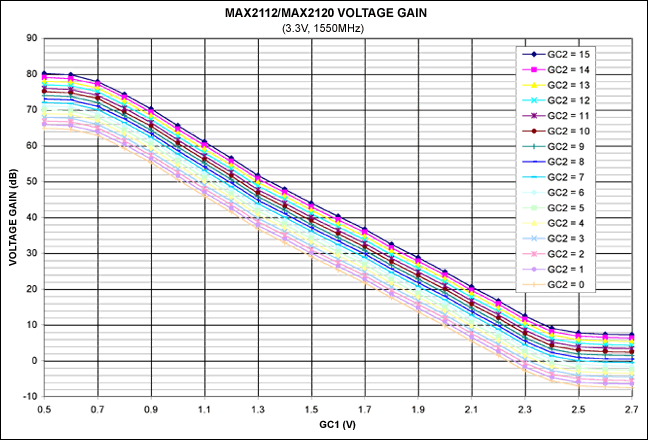Figure 2. Voltage gain vs. GC1 voltage.

Figure 2 shows 72dB of RF gain adjustment provided by the GC1 control voltage. Approximately 15dB of further adjustment is supplied by the baseband gain-control code (GC2). Figures 3 and 4 show the data in greater detail.Figure 3. Voltage gain vs. GC1 voltage (zoomed scale).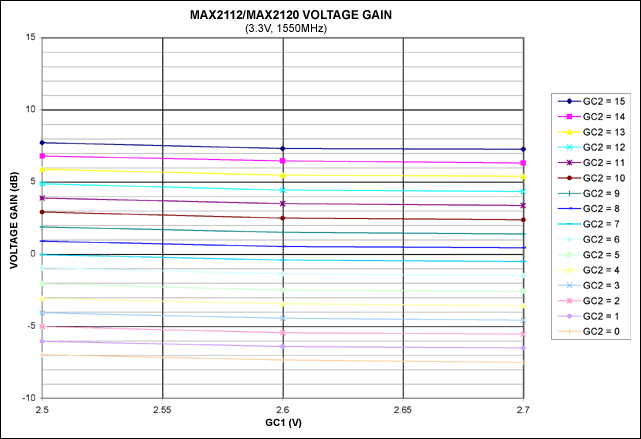Figure 4. Voltage gain vs. GC1 voltage (zoomed scale).

### Input P1dBFigure 5. Input P1dB vs. GC1 voltage.

Figure 5 displays P1dB performance dominated by the RF gain-control voltage GC1, as expected. For GC2 = 8, P1dB ranges from -60dBm to +10dBm, depending on the GC1 setting. A further 10dB of P1dB adjustment is provided by the particular GC2 selection. Figures 6 and 7 show the data in greater detail.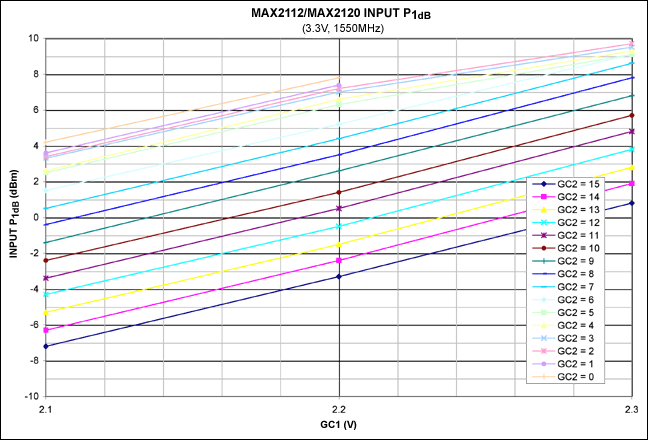Figure 6. Input P1dB vs. GC1 voltage (zoomed scale).Figure 7. Input P1dB vs. GC1 voltage (zoomed scale).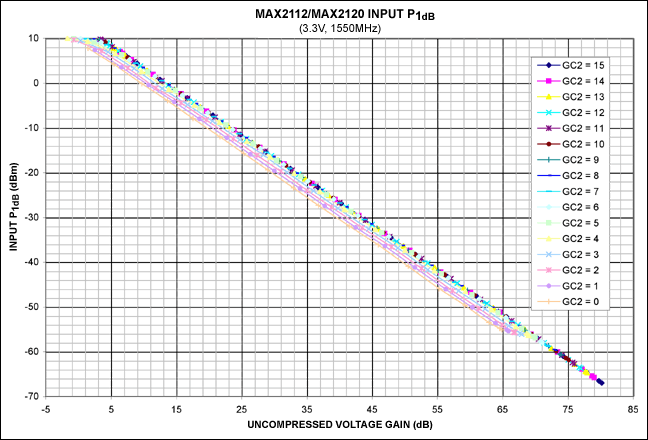Figure 8. Input P1dB vs. uncompressed voltage gain.

Figure 8 illustrates a very small change in P1dB, given a particular uncompressed voltage gain and GC2 in the range of 4 to 15.

### Noise Figure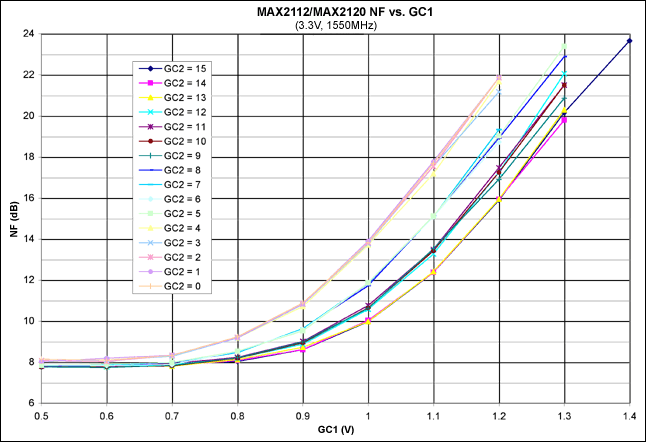Figure 9. NF vs. GC1 voltage.

Figure 9 plots increasing NF as a function of increasing GC1 voltage (decreasing RF gain). Note the four clusters of GC2 codes.Figure 10. NF vs. GC1 voltage (zoomed scale).

At maximum RF gain (GC1 = 0.5V), Figure 10 shows a very small change in NF (0.1dB) for GC2 codes 5 to 15.Figure 11. NF vs. voltage gain.

Figure 11 displays increasing NF as the voltage gain decreases.

### Carrier-to-Noise Ratio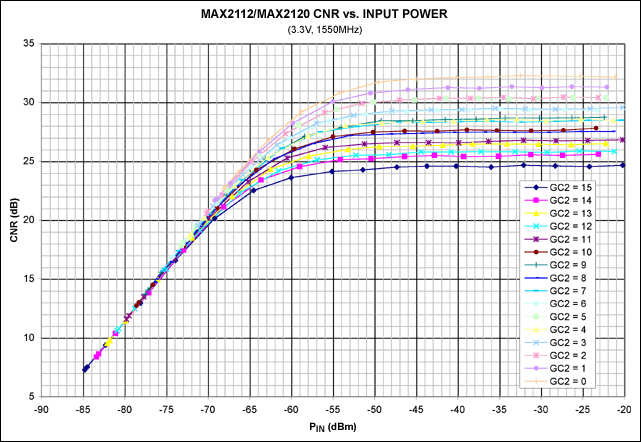Figure 12. CNR vs. input power.

Figure 12 shows the CNR for different input power levels. For each input power and GC2 combination, the GC1 voltage is adjusted for nominal signal level (1VP-P) at the differential baseband output of the tuner. Figure 13 shows the data in greater detail.Figure 13. CNR vs. input power (zoomed scale).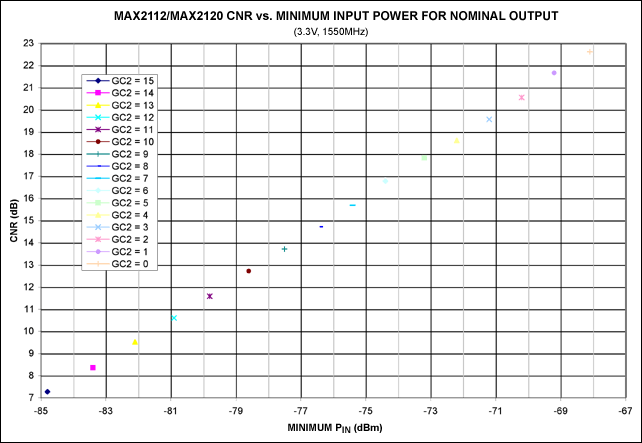Figure 14. CNR vs. minimum input power (PIN).

Figure 14 displays the minimum PIN that provides the nominal output of 1VP-P. These data points are at maximum RF gain. To account for part-to-part variation, 3dB of PIN margin is suggested relative to these typical values. This corresponds to increasing GC2 by 3 codes from the desired minimum PIN point in Figure 14.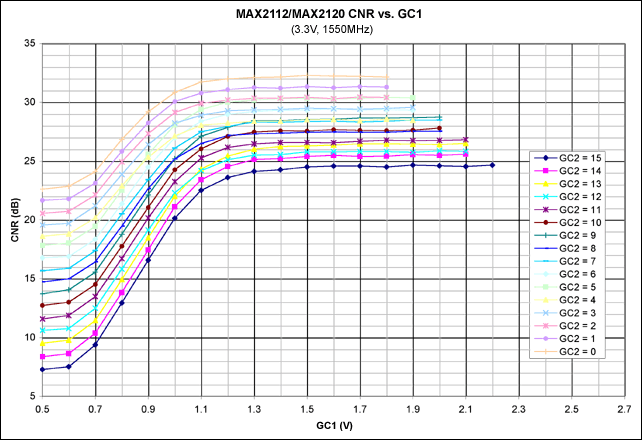Figure 15. CNR vs. GC1.

At maximum RF gain (GC1 = 0.5V), Figure 15 shows CNR varying from 7.3dB to 22.6dB for GC2 codes of 15 and 0, respectively.

### Conclusion

Typical measurement data has been presented that facilitates proper setup of the MAX2112/MAX2120 front-end and back-end gain control.